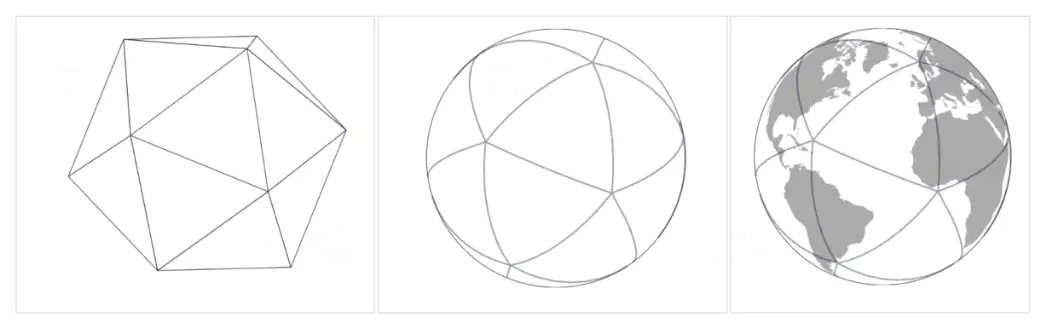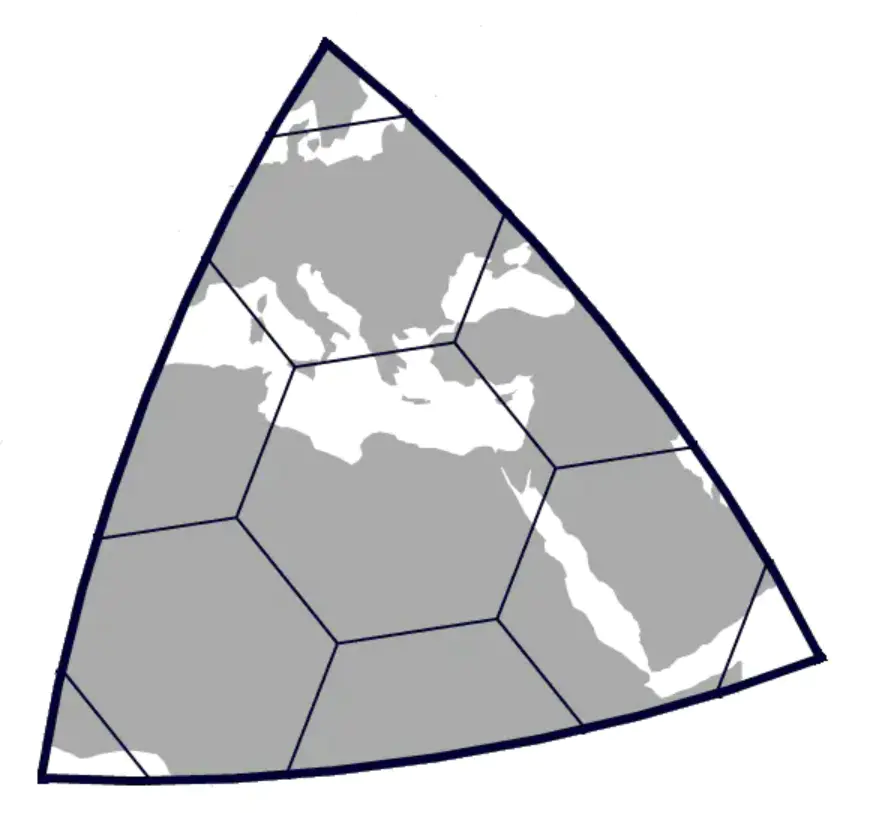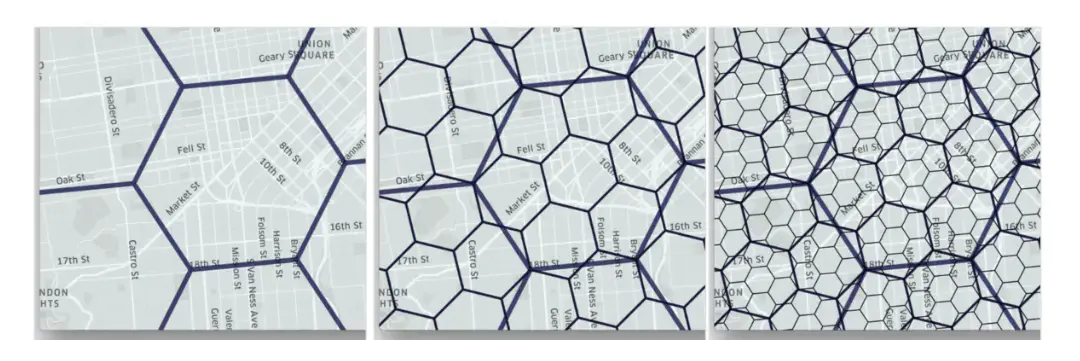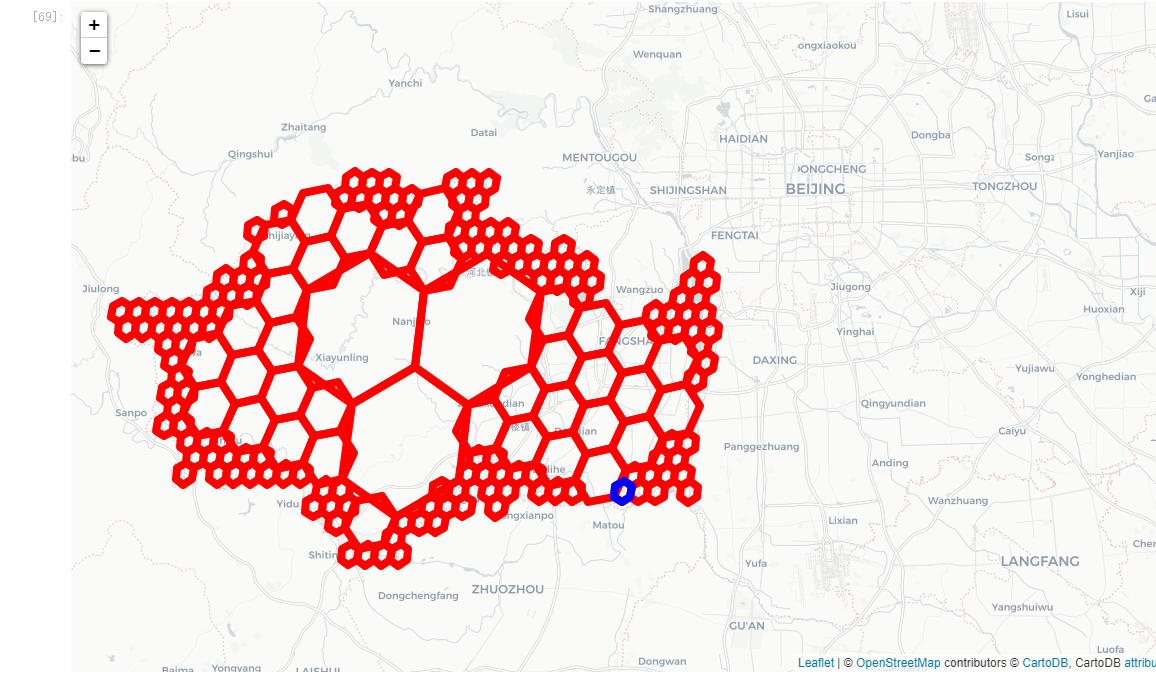## 1. 为什么要用正六边形以及正六边形优点¶

• 首先正六边形相邻单元距离相等，且近似圆，不仅自身近似圆形，贴合密度概念，很适合大多数的汇总分析场景，而且周围相近近似圆形且等距，方便附近查找，阶梯分析等等。

## 2. 全球怎么划分成正六边形¶

• 如果光是正六边形是没办法构成一个球体的，所以将全球分为正二十面体，每个交点有五条边，所以交点处有五边形。• 整体划分• 基本单元

• 并且 H3 支持逐级拆分## 3. 项目中如何使用¶

### 官方示例¶

``````from h3 import h3
import folium

def visualize_hexagons(hexagons, color="red", folium_map=None):
"""
hexagons is a list of hexcluster. Each hexcluster is a list of hexagons.
eg. [[hex1, hex2], [hex3, hex4]]
"""
polylines = []
lat = []
lng = []
for hex in hexagons:
polygons = h3.h3_set_to_multi_polygon([hex], geo_json=False)
# flatten polygons into loops.
outlines = [loop for polygon in polygons for loop in polygon]
polyline = [outline + [outline] for outline in outlines]
lat.extend(map(lambda v:v,polyline))
lng.extend(map(lambda v:v,polyline))
polylines.append(polyline)

if folium_map is None:
m = folium.Map(location=[sum(lat)/len(lat), sum(lng)/len(lng)], zoom_start=13, tiles='cartodbpositron')
else:
m = folium_map
for polyline in polylines:
my_PolyLine=folium.PolyLine(locations=polyline,weight=8,color=color)
return m
``````

### 项目中使用简单示例¶

• 我们使用河北省部分的经纬度聚合得到 H3Id 网格图 , 再通过河北省的任意坐标点来检测，以下是部分代码以及效果示意图
``````h3Ids = ['87318185effffff'] # 这里需要自己聚合生成 H3Ids, 参考官方文档的方法生成
visualize_hexagons(h3Ids)
``````## 4. 结语¶

• 以上就是关于通过 H3 网格聚合 adcode 的简单示例以及效果图，我们可以通过 UberH3 六边形的聚合来达到我们想要的空间效果即空间中的每一个坐标点都可以落到地球中的某一个网格内，经过测试分辨率为 7 的情况下，我们得到的精准 adcode 与高德 api 接口相比，在分辨率为 7 的情况下，能达到 95% 左右，并且对于内存开销也不是很大，中国所有的 H3Ids 存入内存中大概 20M 左右，当然也可以选择更高精度的分辨率前提是某些开销你能接受比如计算机内存开销等等。